# Selina Solutions Concise Maths Class 7 Chapter 8: Percent and Percentage

Selina Solutions Concise Maths Class 7 Chapter 8 Percent and Percentage helps students obtain a clear idea about the fundamental concepts discussed here. Students are advised to solve the textbook problems on a regular basis to score well in the annual exam. For a better understanding of the concepts, students can refer to Selina Solutions Concise Maths Class 7 Chapter 8 Percent and Percentage PDF, from the links provided here.

Chapter 8 helps students understand the steps to be followed in expressing the given statement as percent. Numerous examples present before the problems help students gain confidence in solving tricky problems, with ease.

## Selina Solutions Concise Maths Class 7 Chapter 8: Percent and Percentage Download PDF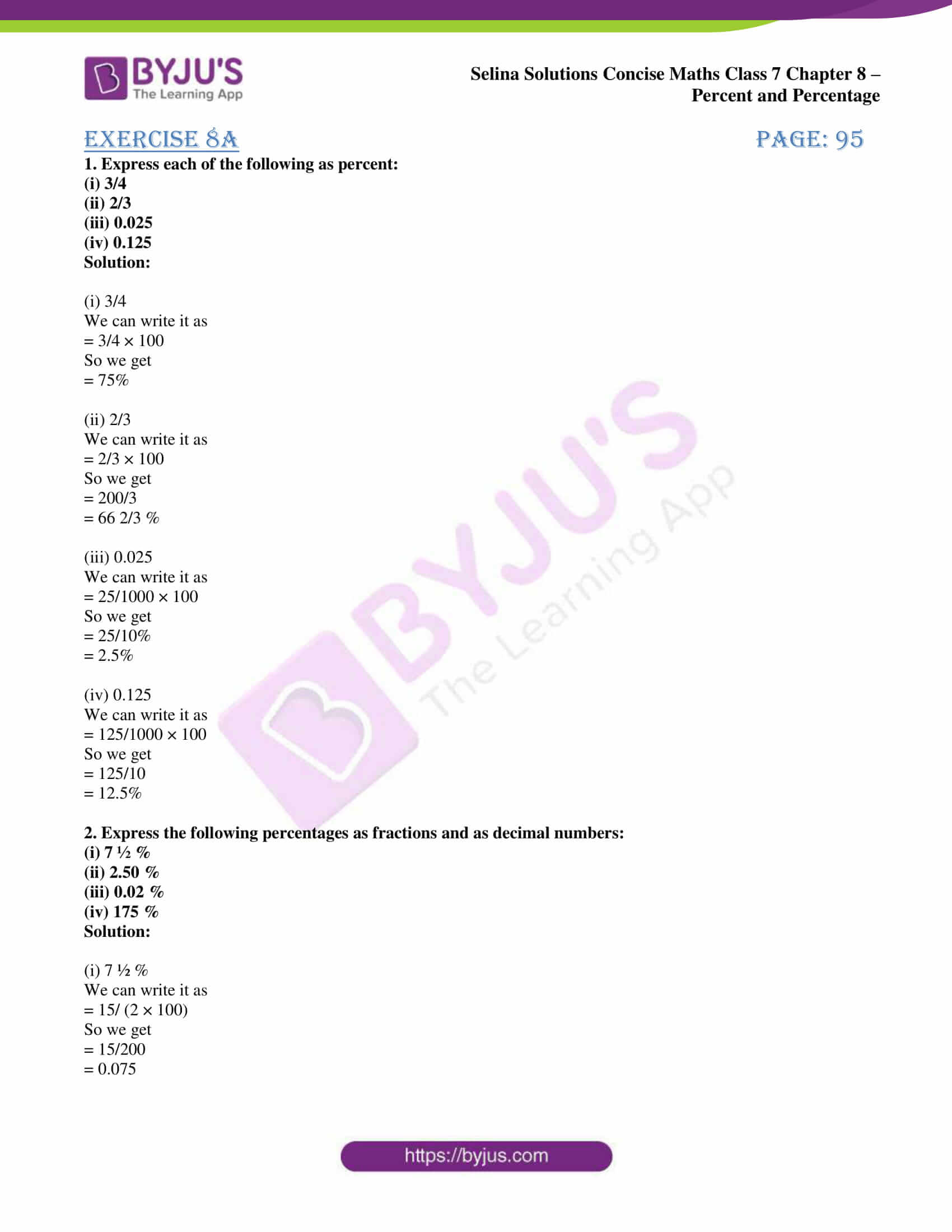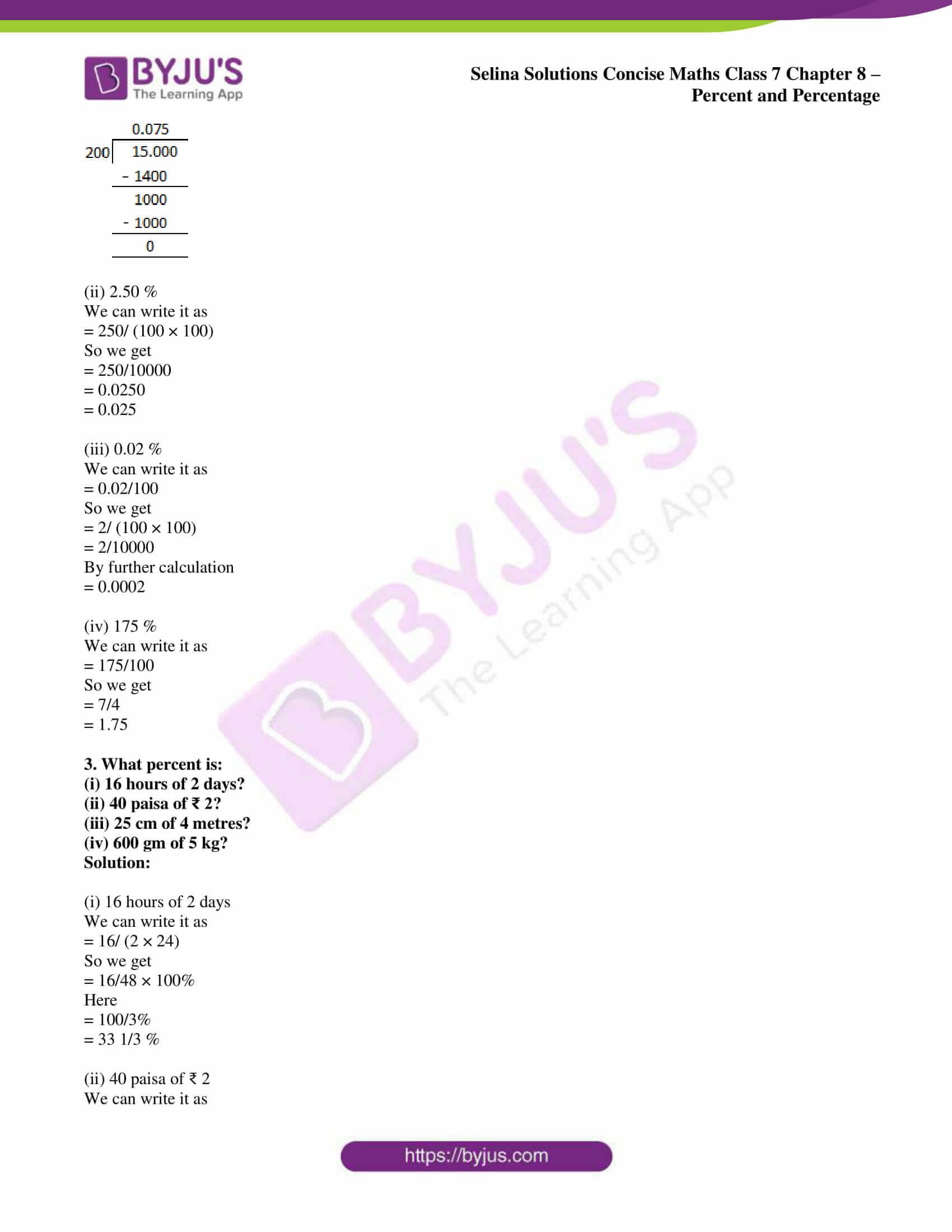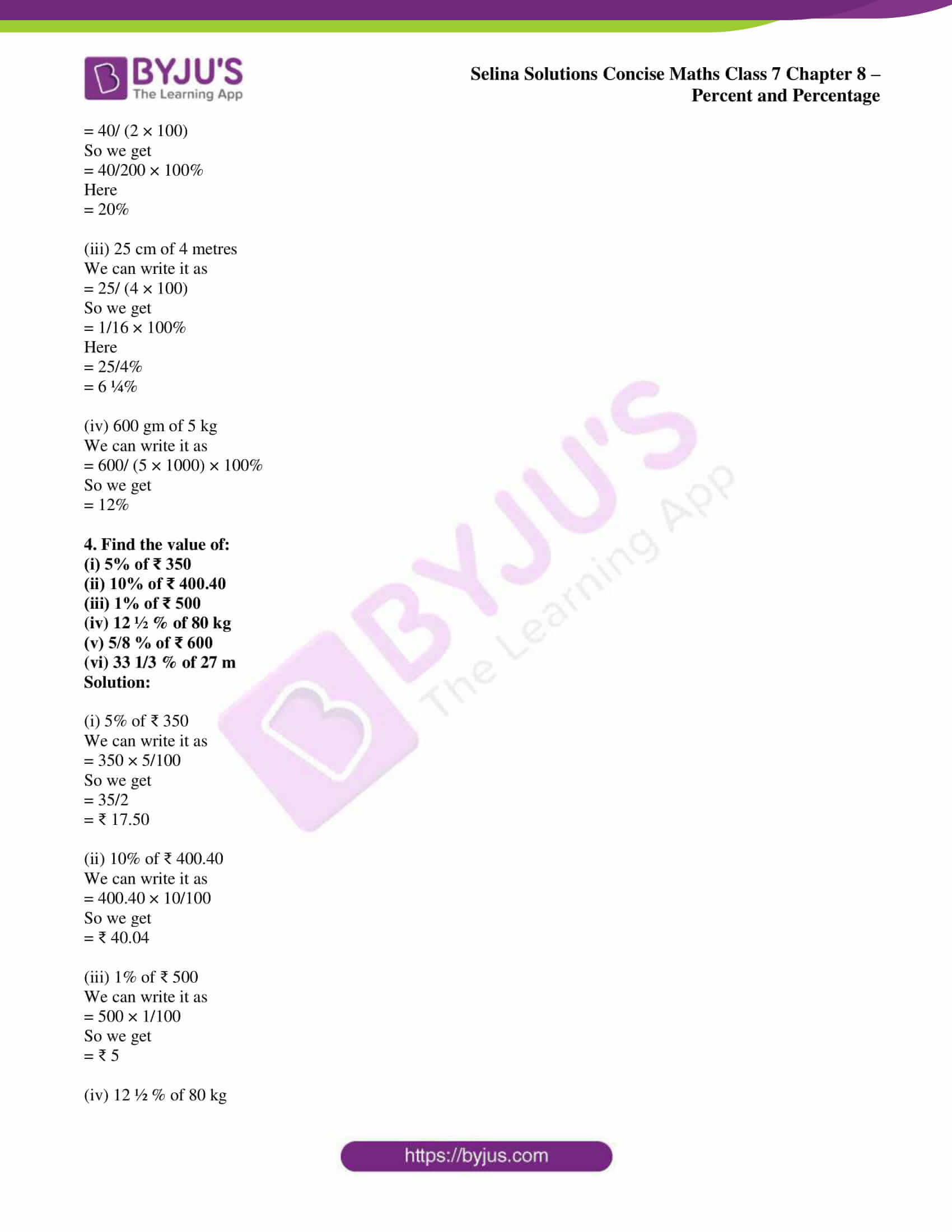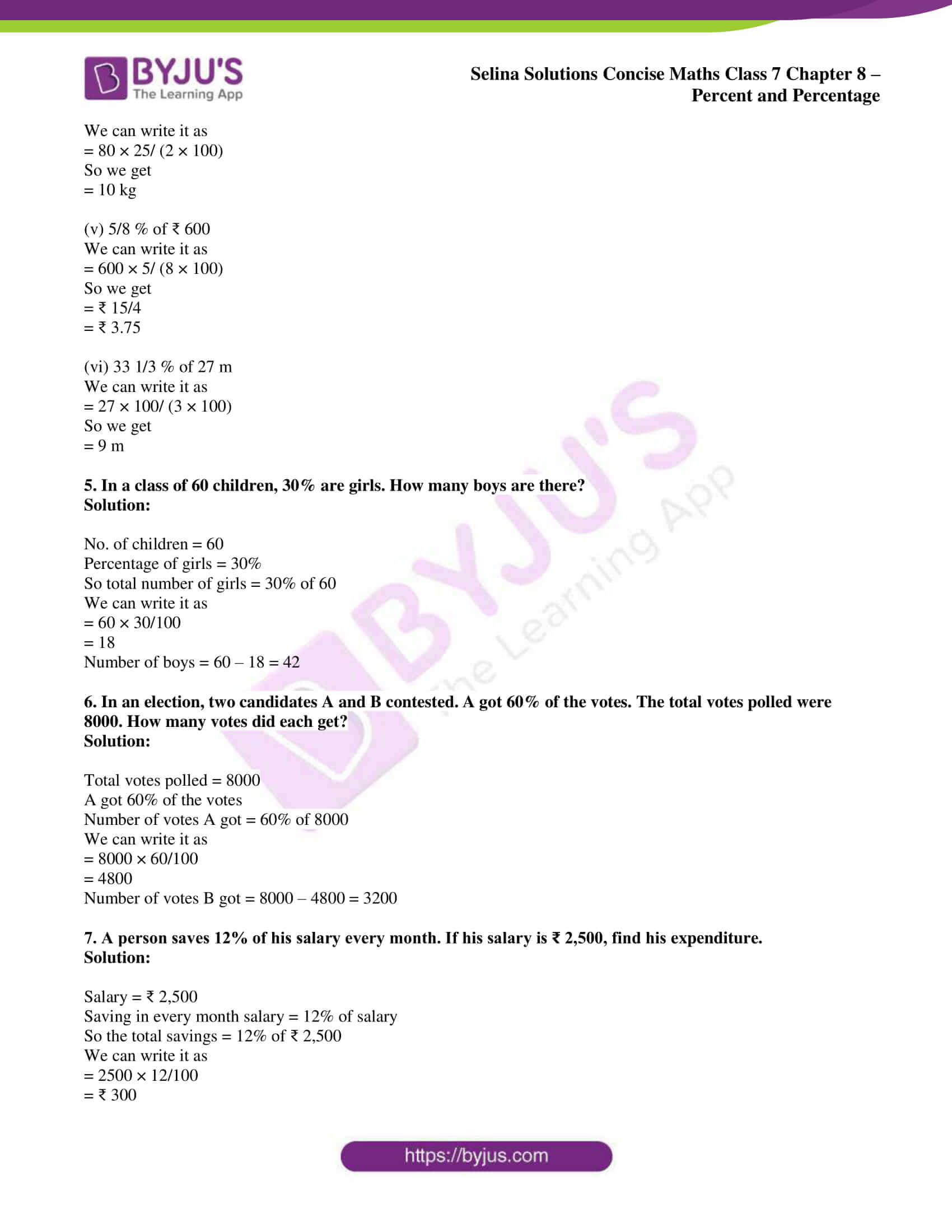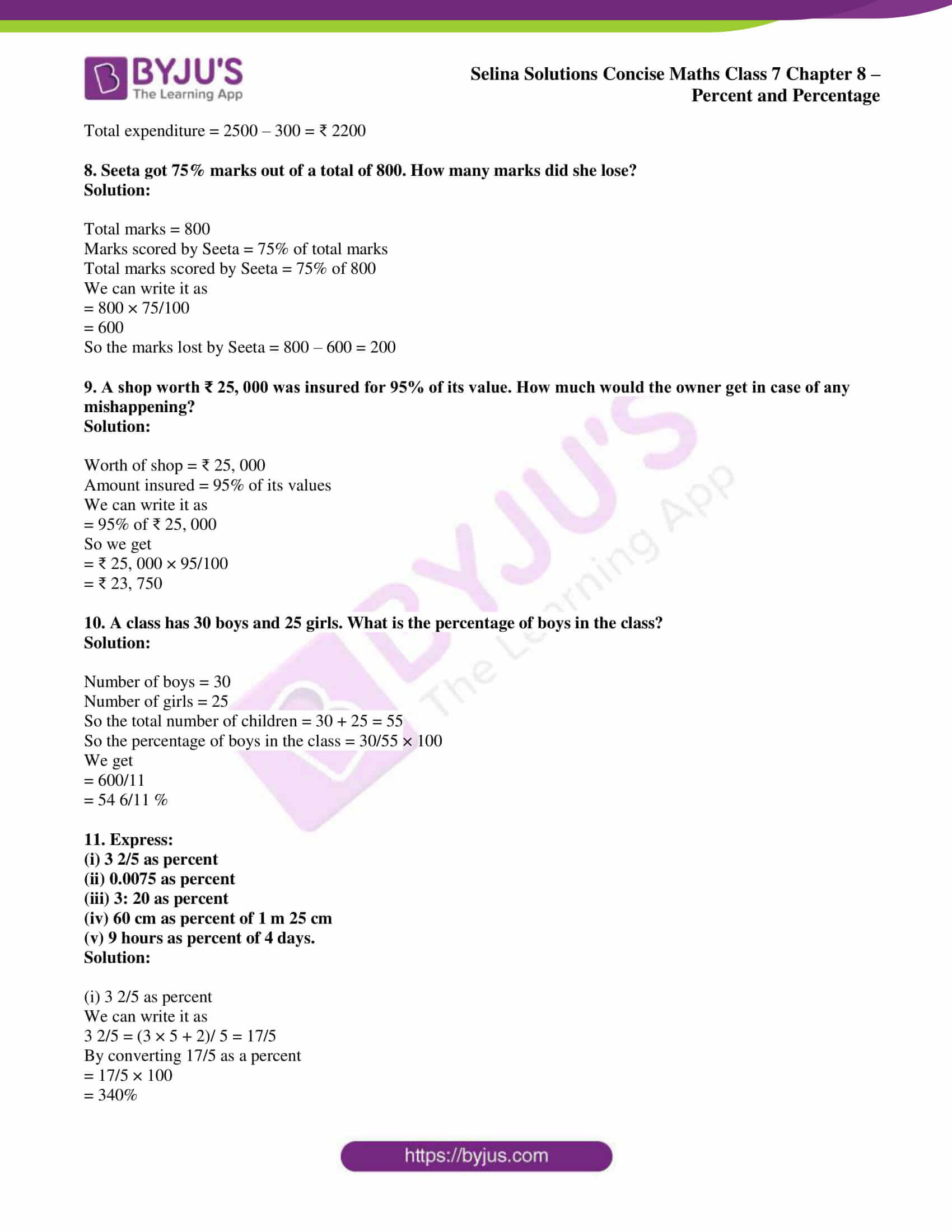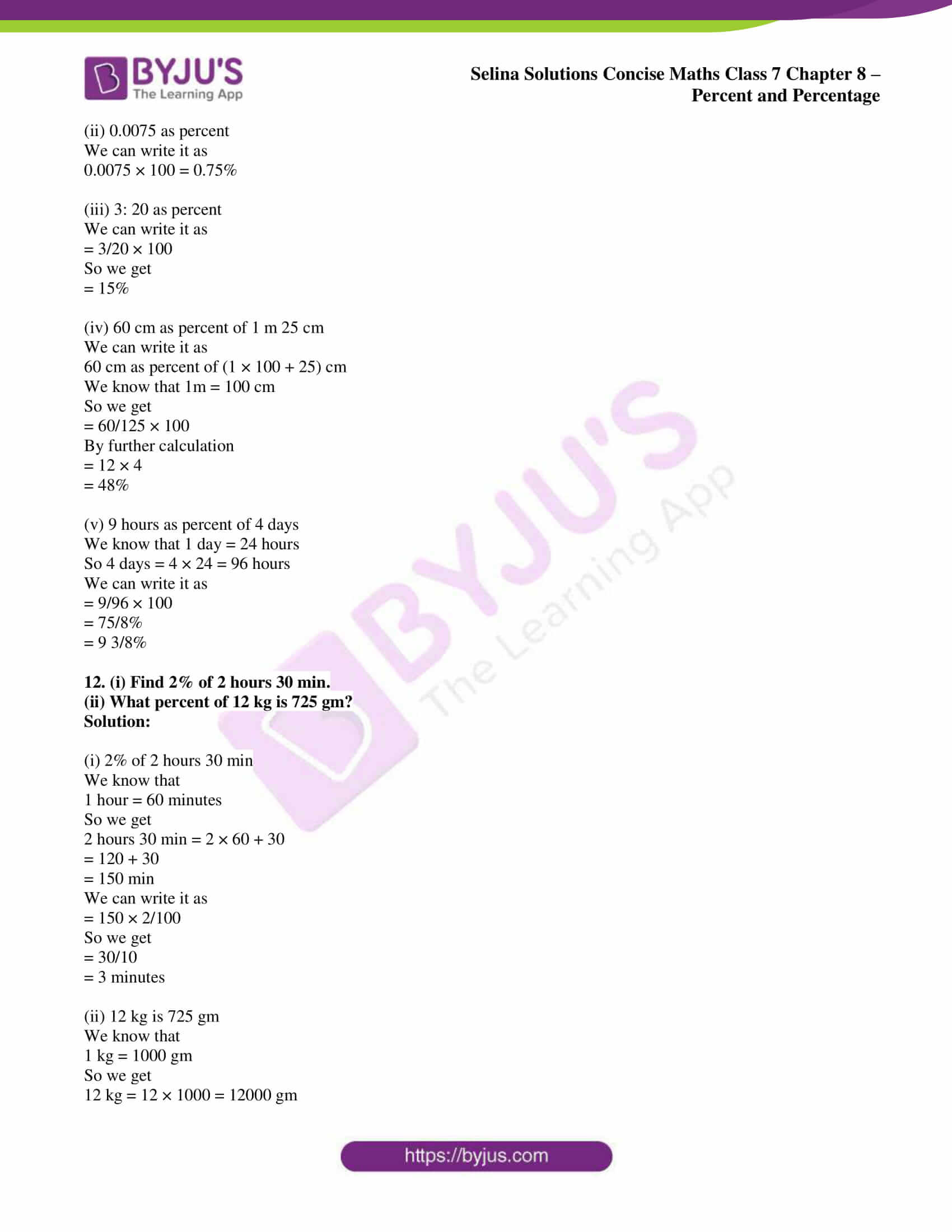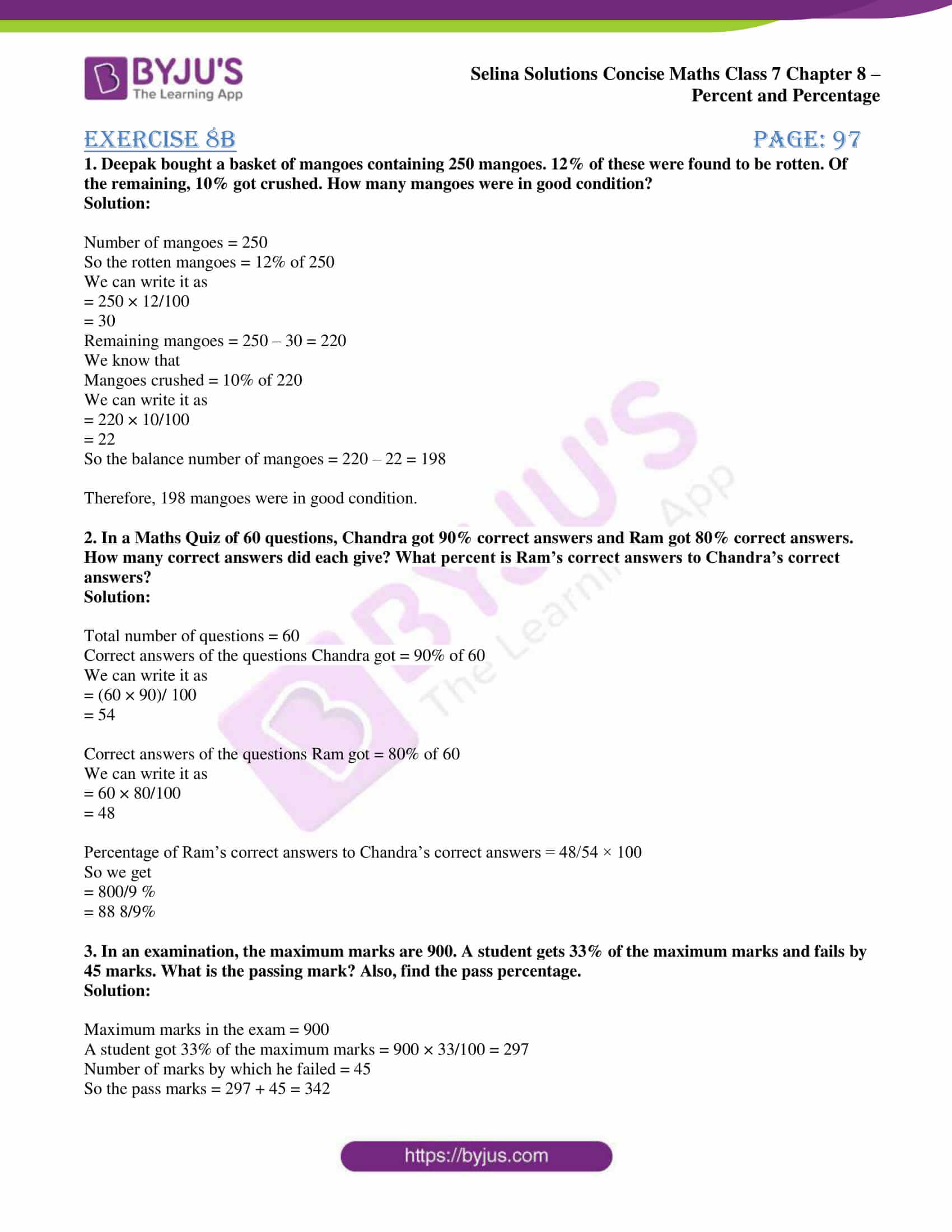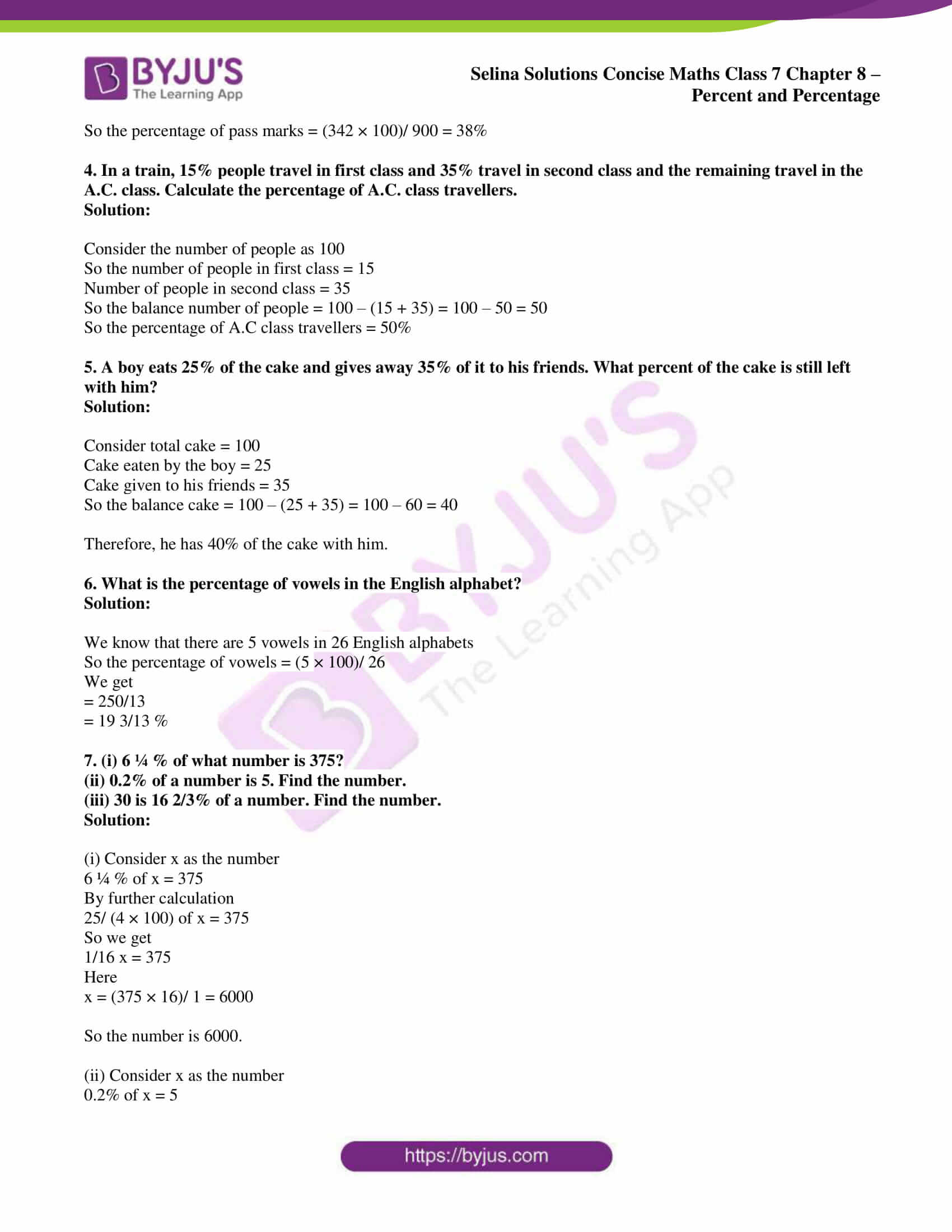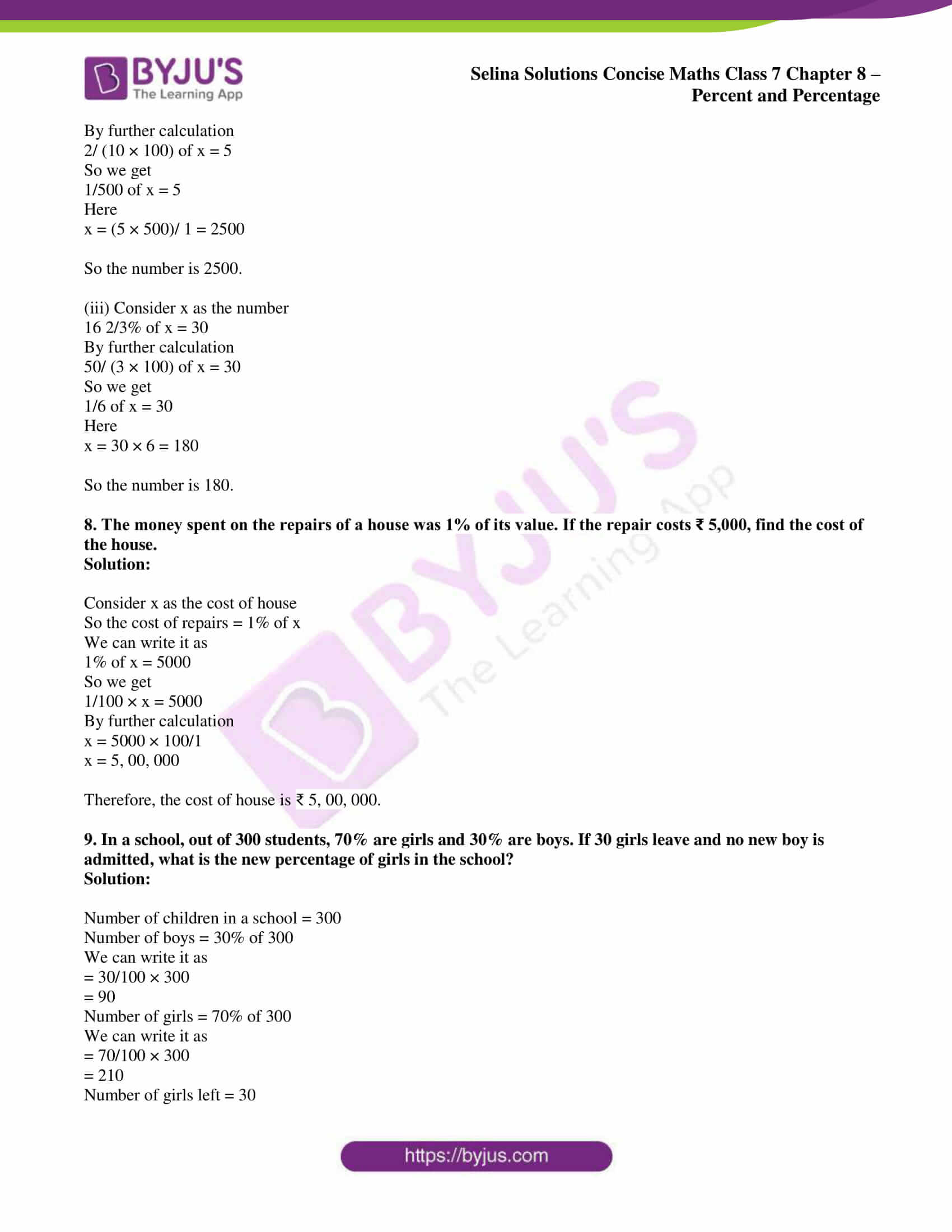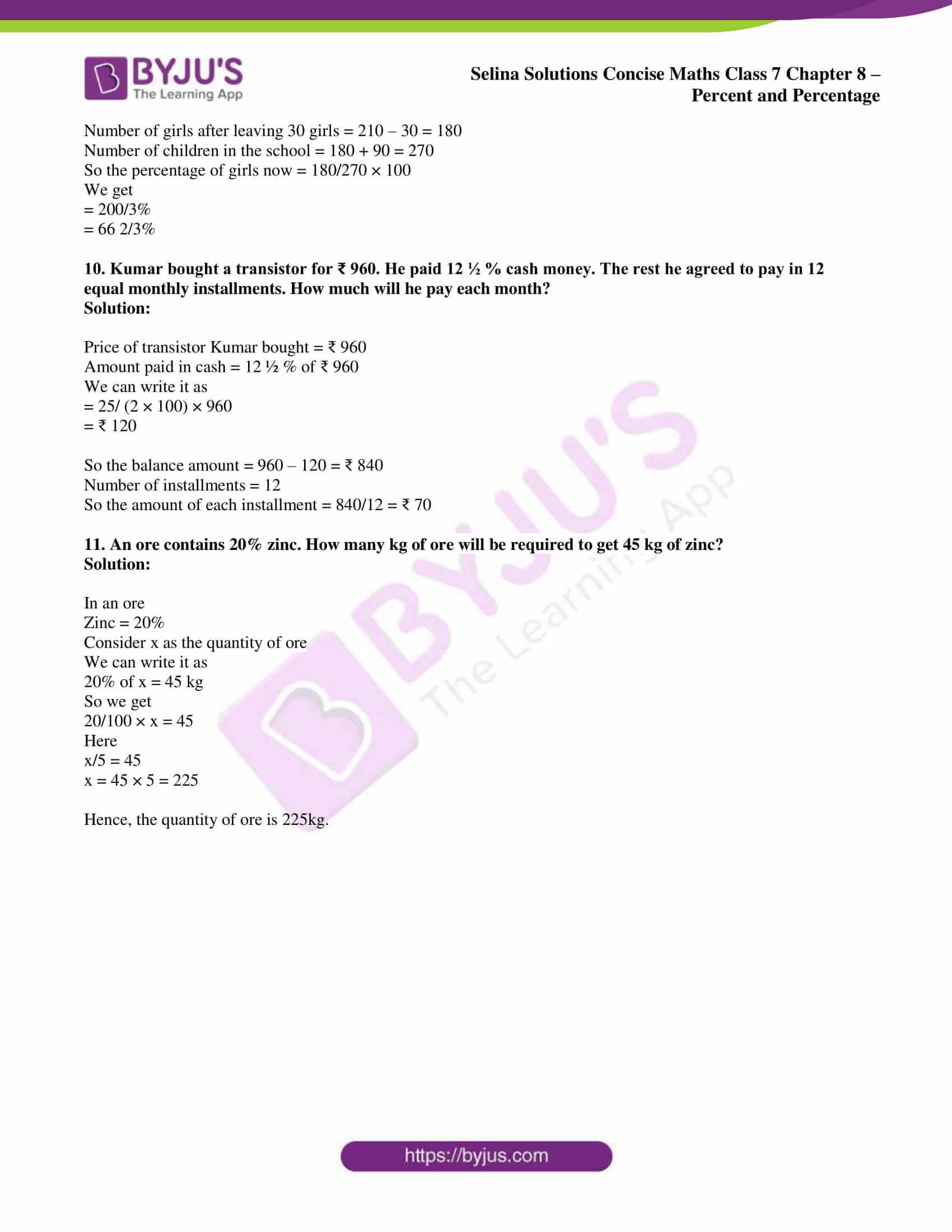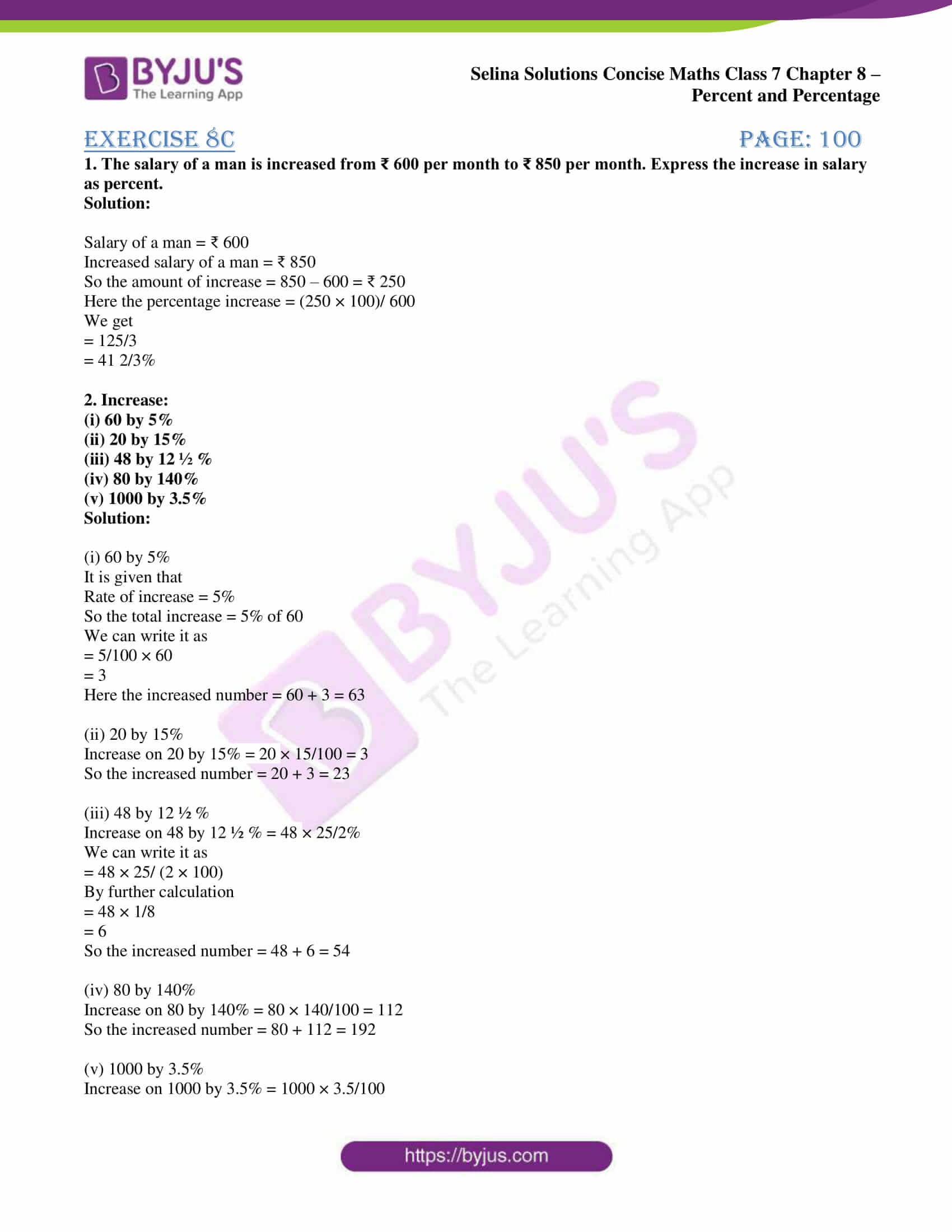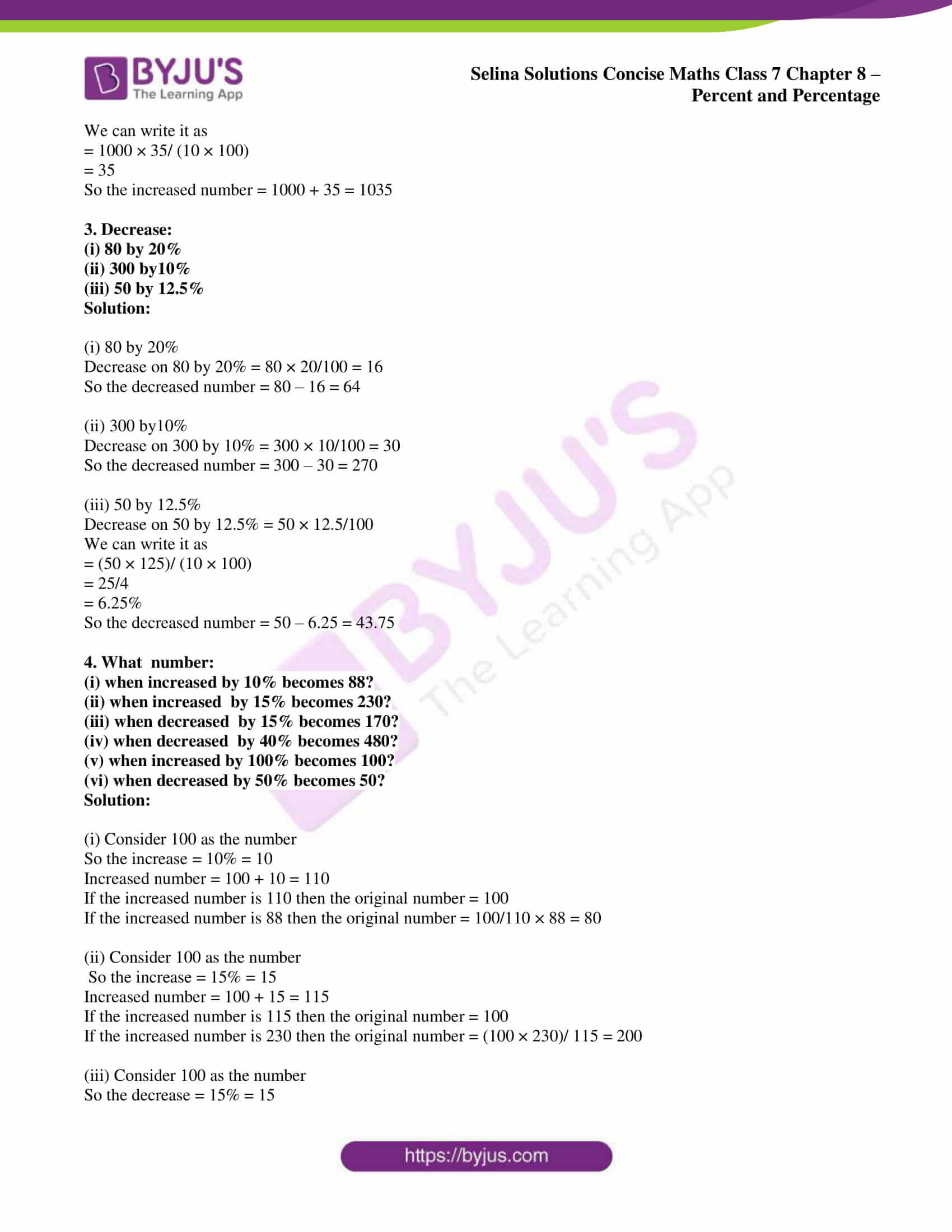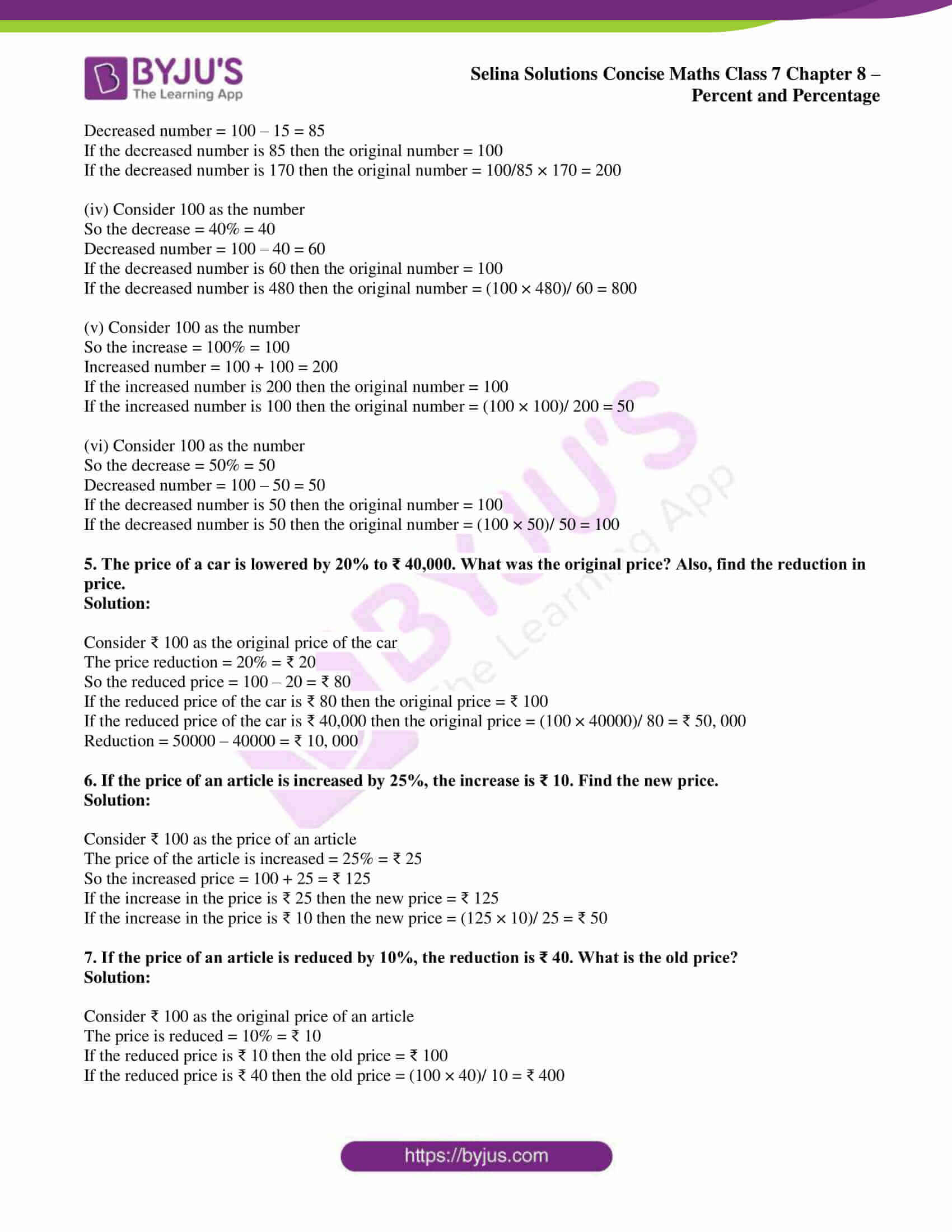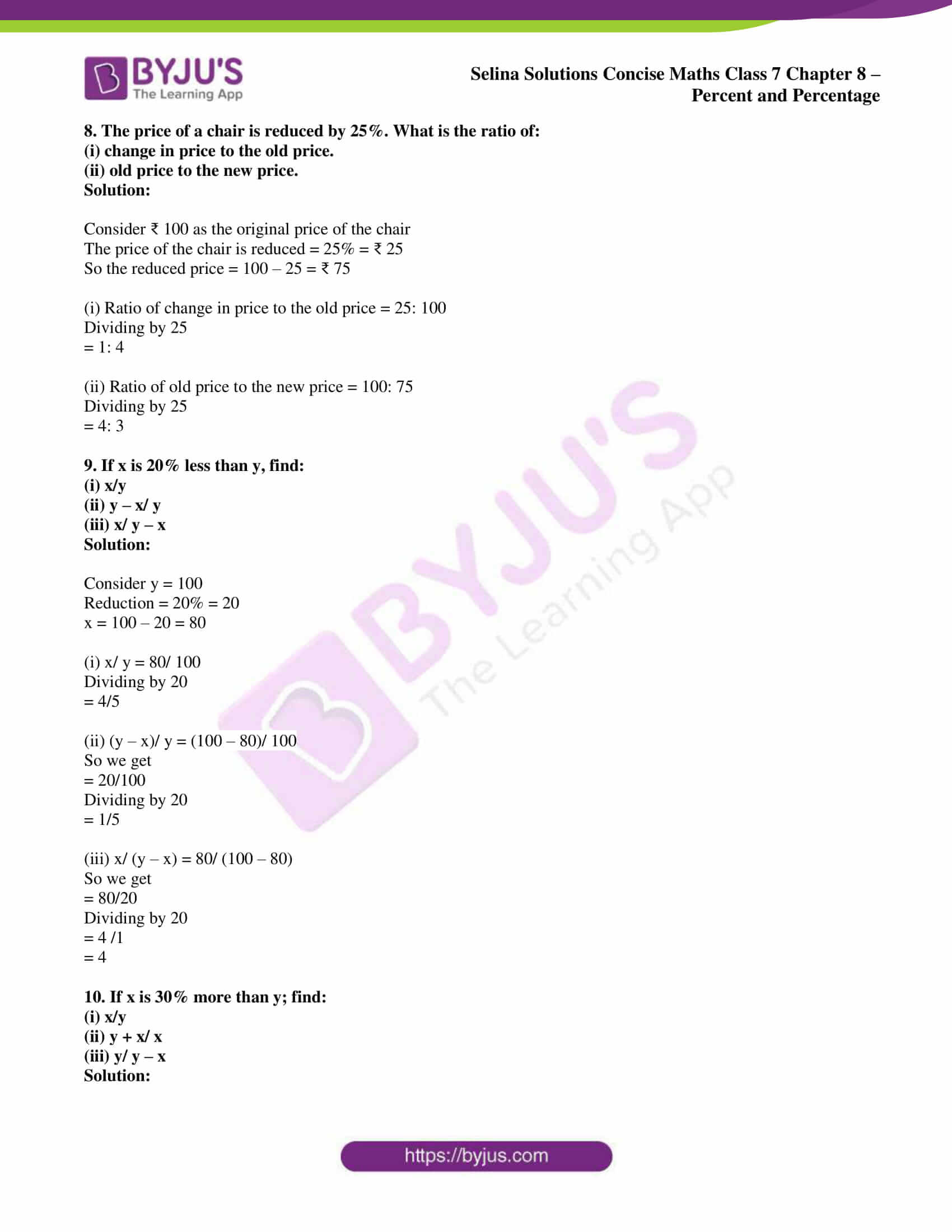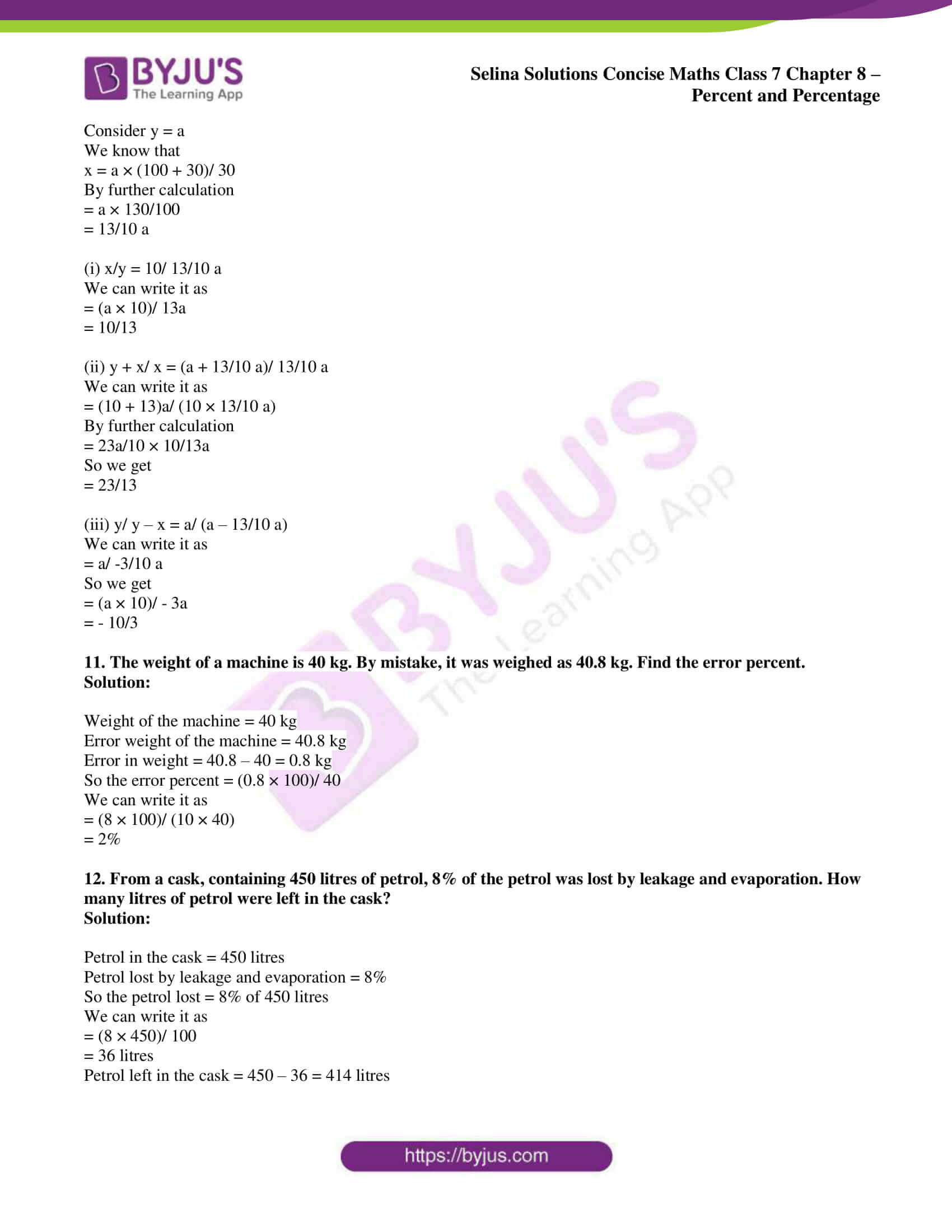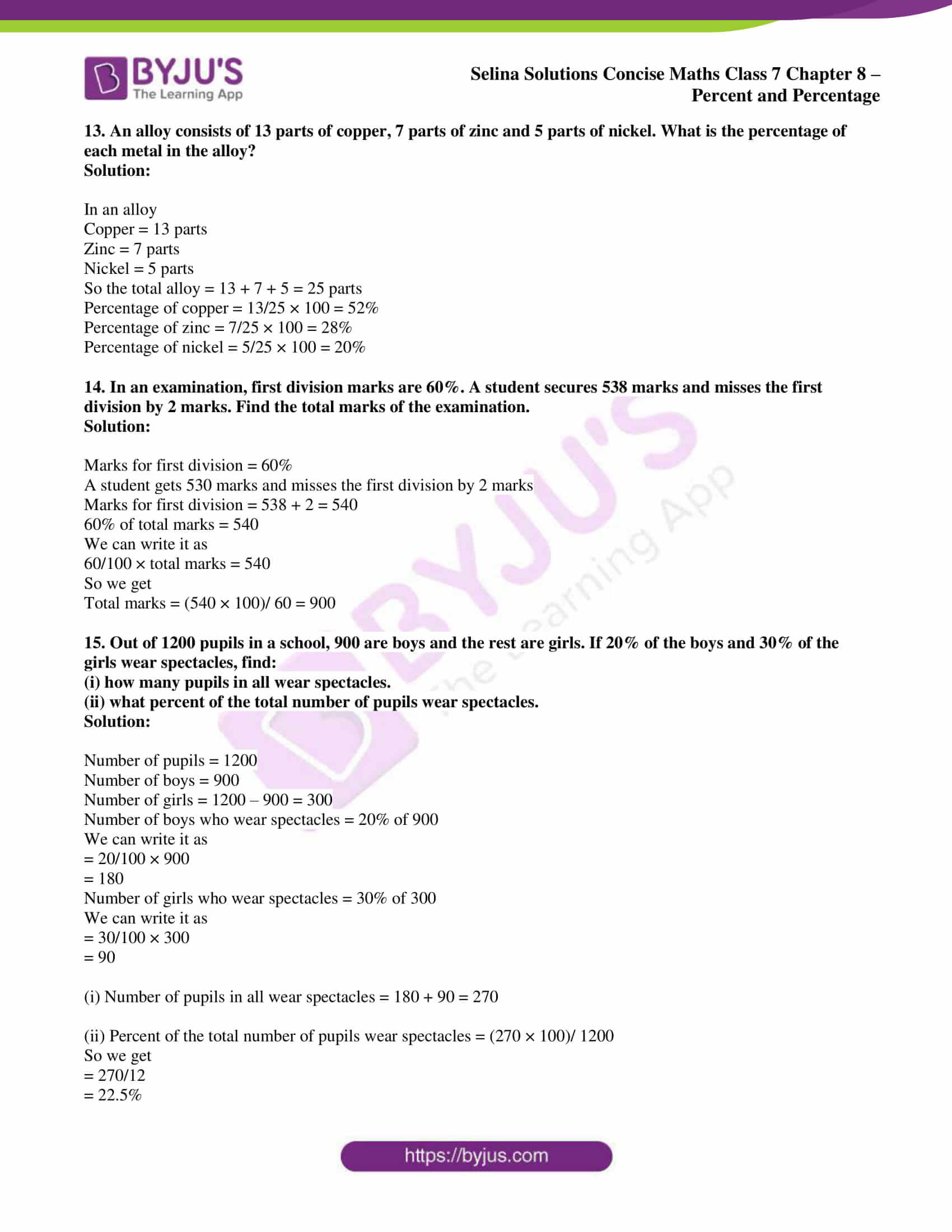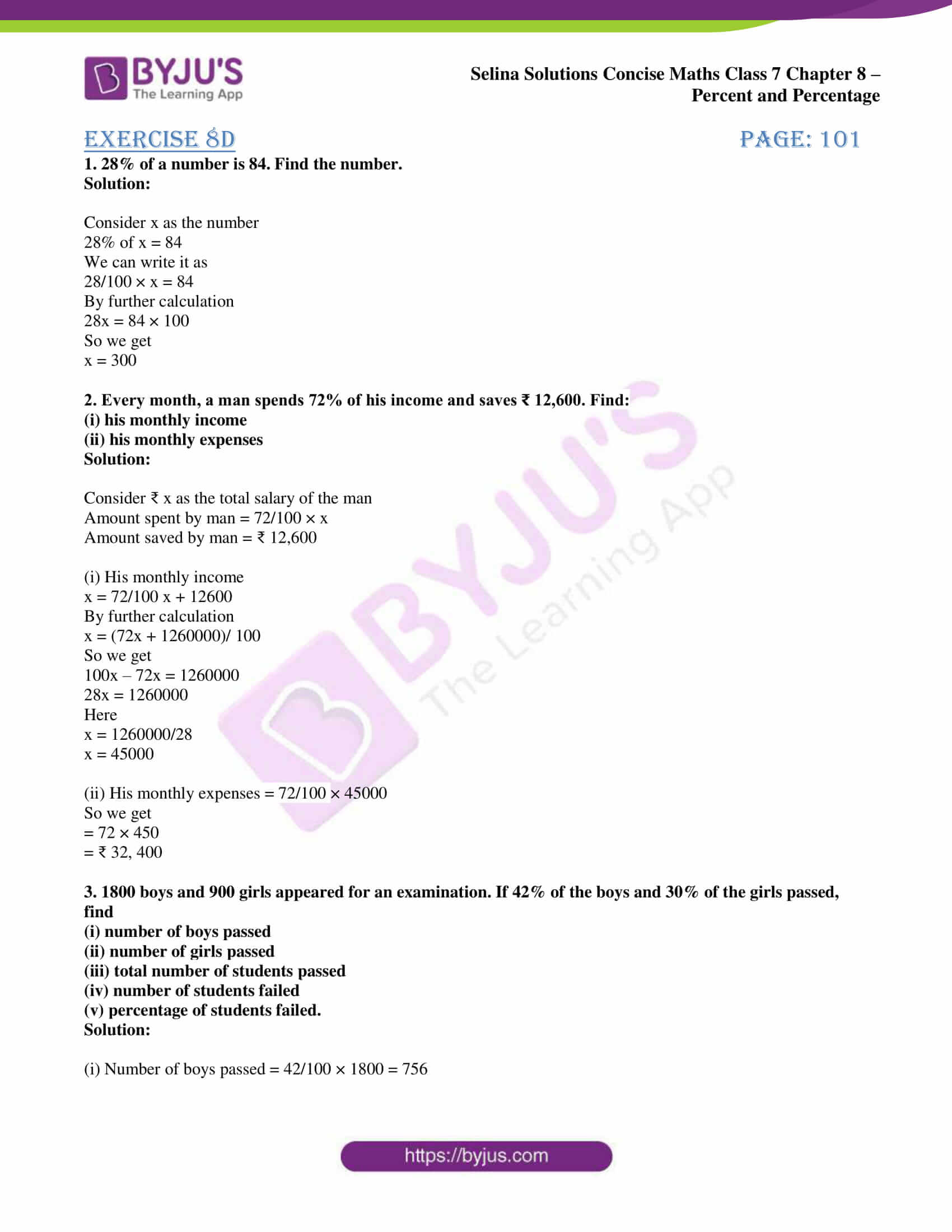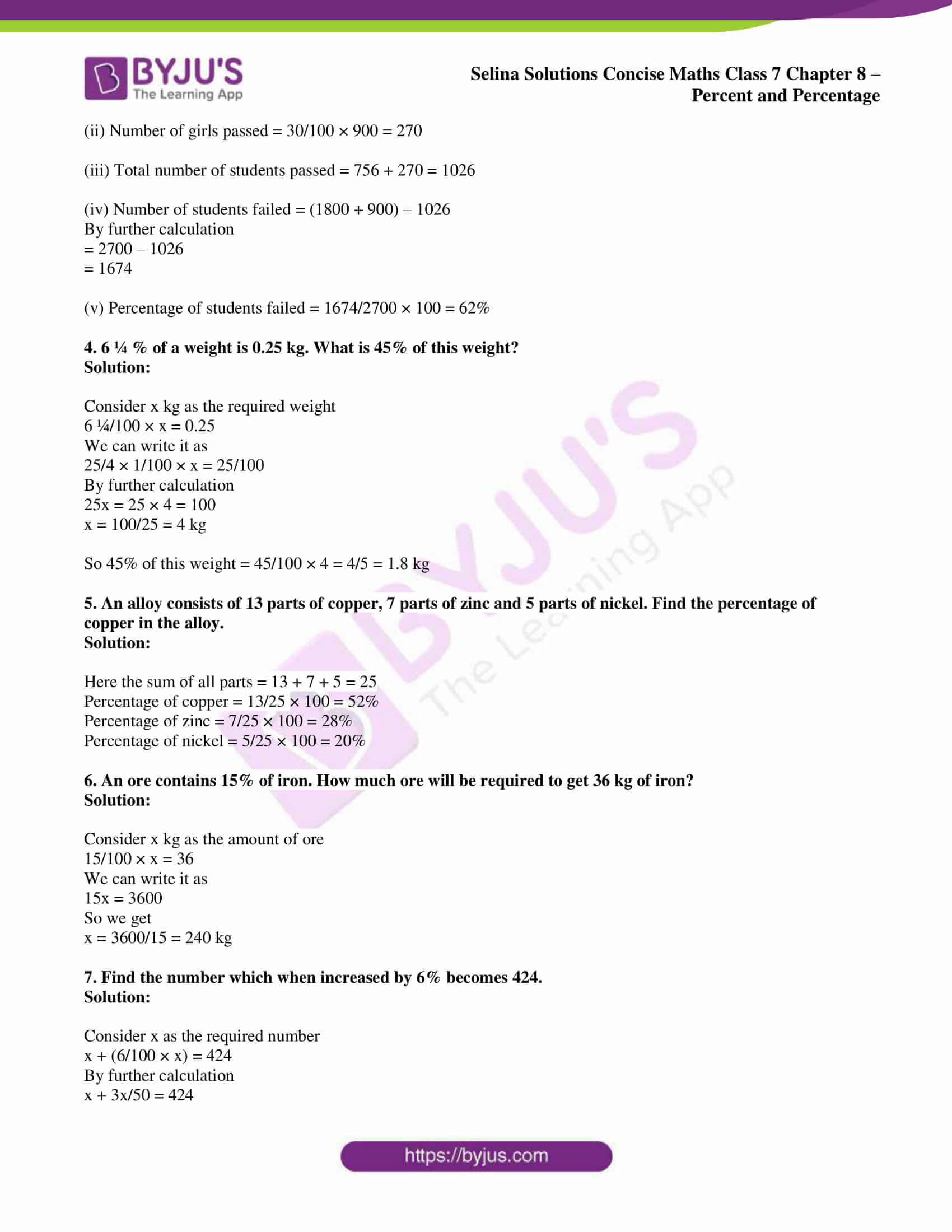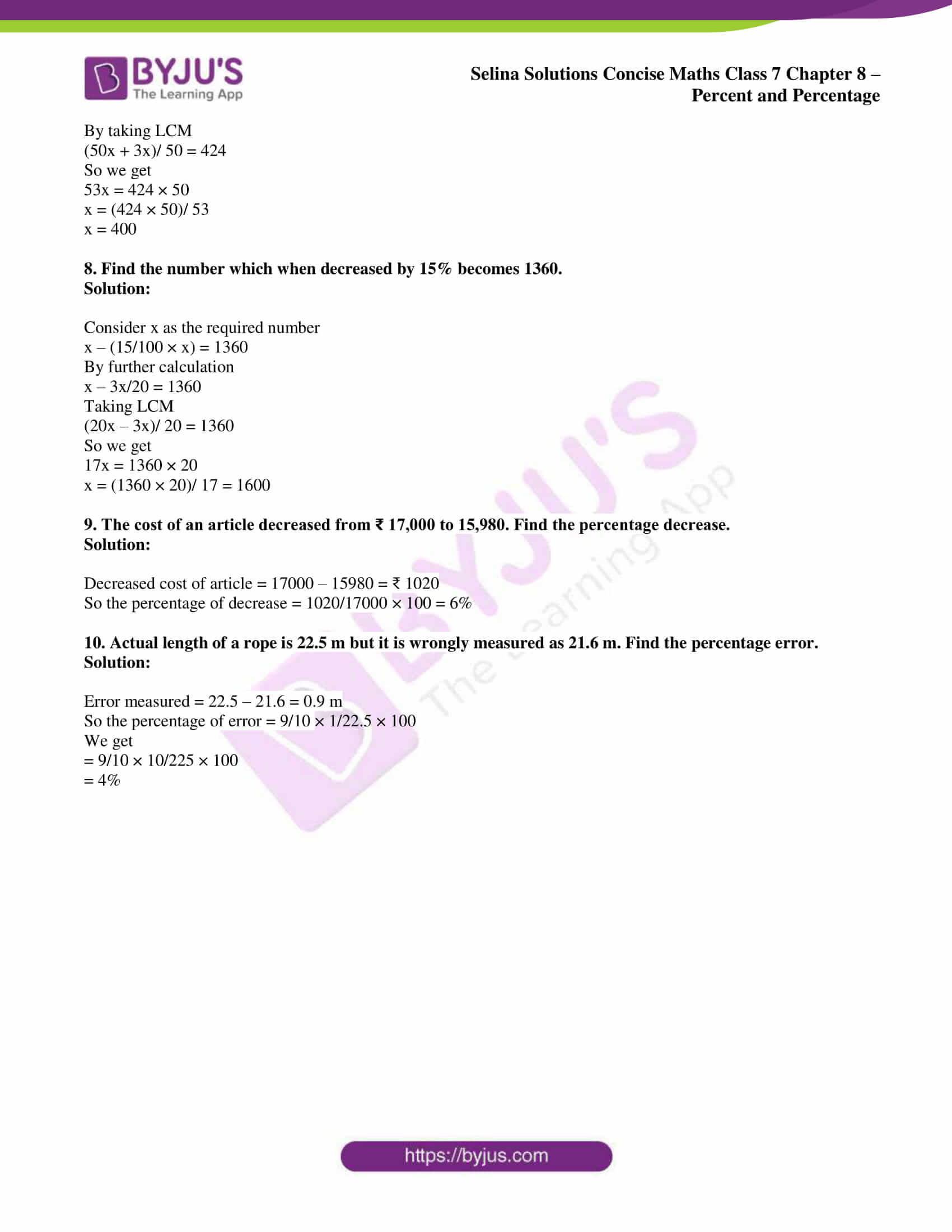## Access Selina Solutions Concise Maths Class 7 Chapter 8: Percent and Percentage

#### Exercise 8A page: 95

1. Express each of the following as percent:

(i) 3/4

(ii) 2/3

(iii) 0.025

(iv) 0.125

Solution:

(i) 3/4

We can write it as

= 3/4 × 100

So we get

= 75%

(ii) 2/3

We can write it as

= 2/3 × 100

So we get

= 200/3

= 66 2/3 %

(iii) 0.025

We can write it as

= 25/1000 × 100

So we get

= 25/10%

= 2.5%

(iv) 0.125

We can write it as

= 125/1000 × 100

So we get

= 125/10

= 12.5%

2. Express the following percentages as fractions and as decimal numbers:

(i) 7 ½ %

(ii) 2.50 %

(iii) 0.02 %

(iv) 175 %

Solution:

(i) 7 ½ %

We can write it as

= 15/ (2 × 100)

So we get

= 15/200

= 0.075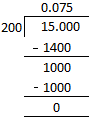(ii) 2.50 %

We can write it as

= 250/ (100 × 100)

So we get

= 250/10000

= 0.0250

= 0.025

(iii) 0.02 %

We can write it as

= 0.02/100

So we get

= 2/ (100 × 100)

= 2/10000

By further calculation

= 0.0002

(iv) 175 %

We can write it as

= 175/100

So we get

= 7/4

= 1.75

3. What percent is:

(i) 16 hours of 2 days?

(ii) 40 paisa of ₹ 2?

(iii) 25 cm of 4 metres?

(iv) 600 gm of 5 kg?

Solution:

(i) 16 hours of 2 days

We can write it as

= 16/ (2 × 24)

So we get

= 16/48 × 100%

Here

= 100/3%

= 33 1/3 %

(ii) 40 paisa of ₹ 2

We can write it as

= 40/ (2 × 100)

So we get

= 40/200 × 100%

Here

= 20%

(iii) 25 cm of 4 metres

We can write it as

= 25/ (4 × 100)

So we get

= 1/16 × 100%

Here

= 25/4%

= 6 ¼%

(iv) 600 gm of 5 kg

We can write it as

= 600/ (5 × 1000) × 100%

So we get

= 12%

4. Find the value of:

(i) 5% of ₹ 350

(ii) 10% of ₹ 400.40

(iii) 1% of ₹ 500

(iv) 12 ½ % of 80 kg

(v) 5/8 % of ₹ 600

(vi) 33 1/3 % of 27 m

Solution:

(i) 5% of ₹ 350

We can write it as

= 350 × 5/100

So we get

= 35/2

= ₹ 17.50

(ii) 10% of ₹ 400.40

We can write it as

= 400.40 × 10/100

So we get

= ₹ 40.04

(iii) 1% of ₹ 500

We can write it as

= 500 × 1/100

So we get

= ₹ 5

(iv) 12 ½ % of 80 kg

We can write it as

= 80 × 25/ (2 × 100)

So we get

= 10 kg

(v) 5/8 % of ₹ 600

We can write it as

= 600 × 5/ (8 × 100)

So we get

= ₹ 15/4

= ₹ 3.75

(vi) 33 1/3 % of 27 m

We can write it as

= 27 × 100/ (3 × 100)

So we get

= 9 m

5. In a class of 60 children, 30% are girls. How many boys are there?

Solution:

No. of children = 60

Percentage of girls = 30%

So total number of girls = 30% of 60

We can write it as

= 60 × 30/100

= 18

Number of boys = 60 – 18 = 42

6. In an election, two candidates A and B contested. A got 60% of the votes. The total votes polled were 8000. How many votes did each get?

Solution:

A got 60% of the votes

Number of votes A got = 60% of 8000

We can write it as

= 8000 × 60/100

= 4800

Number of votes B got = 8000 – 4800 = 3200

7. A person saves 12% of his salary every month. If his salary is ₹ 2,500, find his expenditure.

Solution:

Salary = ₹ 2,500

Saving in every month salary = 12% of salary

So the total savings = 12% of ₹ 2,500

We can write it as

= 2500 × 12/100

= ₹ 300

Total expenditure = 2500 – 300 = ₹ 2200

8. Seeta got 75% marks out of a total of 800. How many marks did she lose?

Solution:

Total marks = 800

Marks scored by Seeta = 75% of total marks

Total marks scored by Seeta = 75% of 800

We can write it as

= 800 × 75/100

= 600

So the marks lost by Seeta = 800 – 600 = 200

9. A shop worth ₹ 25, 000 was insured for 95% of its value. How much would the owner get in case of any mishappening?

Solution:

Worth of shop = ₹ 25, 000

Amount insured = 95% of its values

We can write it as

= 95% of ₹ 25, 000

So we get

= ₹ 25, 000 × 95/100

= ₹ 23, 750

10. A class has 30 boys and 25 girls. What is the percentage of boys in the class?

Solution:

Number of boys = 30

Number of girls = 25

So the total number of children = 30 + 25 = 55

So the percentage of boys in the class = 30/55 × 100

We get

= 600/11

= 54 6/11 %

11. Express:

(i) 3 2/5 as percent

(ii) 0.0075 as percent

(iii) 3: 20 as percent

(iv) 60 cm as percent of 1 m 25 cm

(v) 9 hours as percent of 4 days.

Solution:

(i) 3 2/5 as percent

We can write it as

3 2/5 = (3 × 5 + 2)/ 5 = 17/5

By converting 17/5 as a percent

= 17/5 × 100

= 340%

(ii) 0.0075 as percent

We can write it as

0.0075 × 100 = 0.75%

(iii) 3: 20 as percent

We can write it as

= 3/20 × 100

So we get

= 15%

(iv) 60 cm as percent of 1 m 25 cm

We can write it as

60 cm as percent of (1 × 100 + 25) cm

We know that 1m = 100 cm

So we get

= 60/125 × 100

By further calculation

= 12 × 4

= 48%

(v) 9 hours as percent of 4 days

We know that 1 day = 24 hours

So 4 days = 4 × 24 = 96 hours

We can write it as

= 9/96 × 100

= 75/8%

= 9 3/8%

12. (i) Find 2% of 2 hours 30 min.

(ii) What percent of 12 kg is 725 gm?

Solution:

(i) 2% of 2 hours 30 min

We know that

1 hour = 60 minutes

So we get

2 hours 30 min = 2 × 60 + 30

= 120 + 30

= 150 min

We can write it as

= 150 × 2/100

So we get

= 30/10

= 3 minutes

(ii) 12 kg is 725 gm

We know that

1 kg = 1000 gm

So we get

12 kg = 12 × 1000 = 12000 gm

We can write it as

= 725/12000 × 100

So we get

= 725/120

= 145/24%

= 6 1/24%

#### Exercise 8B page: 97

1. Deepak bought a basket of mangoes containing 250 mangoes. 12% of these were found to be rotten. Of the remaining, 10% got crushed. How many mangoes were in good condition?

Solution:

Number of mangoes = 250

So the rotten mangoes = 12% of 250

We can write it as

= 250 × 12/100

= 30

Remaining mangoes = 250 – 30 = 220

We know that

Mangoes crushed = 10% of 220

We can write it as

= 220 × 10/100

= 22

So the balance number of mangoes = 220 – 22 = 198

Therefore, 198 mangoes were in good condition.

2. In a Maths Quiz of 60 questions, Chandra got 90% correct answers and Ram got 80% correct answers. How many correct answers did each give? What percent is Ram’s correct answers to Chandra’s correct answers?

Solution:

Total number of questions = 60

Correct answers of the questions Chandra got = 90% of 60

We can write it as

= (60 × 90)/ 100

= 54

Correct answers of the questions Ram got = 80% of 60

We can write it as

= 60 × 80/100

= 48

Percentage of Ram’s correct answers to Chandra’s correct answers = 48/54 × 100

So we get

= 800/9 %

= 88 8/9%

3. In an examination, the maximum marks are 900. A student gets 33% of the maximum marks and fails by 45 marks. What is the passing mark? Also, find the pass percentage.

Solution:

Maximum marks in the exam = 900

A student got 33% of the maximum marks = 900 × 33/100 = 297

Number of marks by which he failed = 45

So the pass marks = 297 + 45 = 342

So the percentage of pass marks = (342 × 100)/ 900 = 38%

4. In a train, 15% people travel in first class and 35% travel in second class and the remaining travel in the A.C. class. Calculate the percentage of A.C. class travellers.

Solution:

Consider the number of people as 100

So the number of people in first class = 15

Number of people in second class = 35

So the balance number of people = 100 – (15 + 35) = 100 – 50 = 50

So the percentage of A.C class travellers = 50%

5. A boy eats 25% of the cake and gives away 35% of it to his friends. What percent of the cake is still left with him?

Solution:

Consider total cake = 100

Cake eaten by the boy = 25

Cake given to his friends = 35

So the balance cake = 100 – (25 + 35) = 100 – 60 = 40

Therefore, he has 40% of the cake with him.

6. What is the percentage of vowels in the English alphabet?

Solution:

We know that there are 5 vowels in 26 English alphabets

So the percentage of vowels = (5 × 100)/ 26

We get

= 250/13

= 19 3/13 %

7. (i) 6 ¼ % of what number is 375?

(ii) 0.2% of a number is 5. Find the number.

(iii) 30 is 16 2/3% of a number. Find the number.

Solution:

(i) Consider x as the number

6 ¼ % of x = 375

By further calculation

25/ (4 × 100) of x = 375

So we get

1/16 x = 375

Here

x = (375 × 16)/ 1 = 6000

So the number is 6000.

(ii) Consider x as the number

0.2% of x = 5

By further calculation

2/ (10 × 100) of x = 5

So we get

1/500 of x = 5

Here

x = (5 × 500)/ 1 = 2500

So the number is 2500.

(iii) Consider x as the number

16 2/3% of x = 30

By further calculation

50/ (3 × 100) of x = 30

So we get

1/6 of x = 30

Here

x = 30 × 6 = 180

So the number is 180.

8. The money spent on the repairs of a house was 1% of its value. If the repair costs ₹ 5,000, find the cost of the house.

Solution:

Consider x as the cost of house

So the cost of repairs = 1% of x

We can write it as

1% of x = 5000

So we get

1/100 × x = 5000

By further calculation

x = 5000 × 100/1

x = 5, 00, 000

Therefore, the cost of house is ₹ 5, 00, 000.

9. In a school, out of 300 students, 70% are girls and 30% are boys. If 30 girls leave and no new boy is admitted, what is the new percentage of girls in the school?

Solution:

Number of children in a school = 300

Number of boys = 30% of 300

We can write it as

= 30/100 × 300

= 90

Number of girls = 70% of 300

We can write it as

= 70/100 × 300

= 210

Number of girls left = 30

Number of girls after leaving 30 girls = 210 – 30 = 180

Number of children in the school = 180 + 90 = 270

So the percentage of girls now = 180/270 × 100

We get

= 200/3%

= 66 2/3%

10. Kumar bought a transistor for ₹ 960. He paid 12 ½ % cash money. The rest he agreed to pay in 12 equal monthly installments. How much will he pay each month?

Solution:

Price of transistor Kumar bought = ₹ 960

Amount paid in cash = 12 ½ % of ₹ 960

We can write it as

= 25/ (2 × 100) × 960

= ₹ 120

So the balance amount = 960 – 120 = ₹ 840

Number of installments = 12

So the amount of each installment = 840/12 = ₹ 70

11. An ore contains 20% zinc. How many kg of ore will be required to get 45 kg of zinc?

Solution:

In an ore

Zinc = 20%

Consider x as the quantity of ore

We can write it as

20% of x = 45 kg

So we get

20/100 × x = 45

Here

x/5 = 45

x = 45 × 5 = 225

Hence, the quantity of ore is 225kg.

#### Exercise 8C page: 100

1. The salary of a man is increased from ₹ 600 per month to ₹ 850 per month. Express the increase in salary as percent.

Solution:

Salary of a man = ₹ 600

Increased salary of a man = ₹ 850

So the amount of increase = 850 – 600 = ₹ 250

Here the percentage increase = (250 × 100)/ 600

We get

= 125/3

= 41 2/3%

2. Increase:

(i) 60 by 5%

(ii) 20 by 15%

(iii) 48 by 12 ½ %

(iv) 80 by 140%

(v) 1000 by 3.5%

Solution:

(i) 60 by 5%

It is given that

Rate of increase = 5%

So the total increase = 5% of 60

We can write it as

= 5/100 × 60

= 3

Here the increased number = 60 + 3 = 63

(ii) 20 by 15%

Increase on 20 by 15% = 20 × 15/100 = 3

So the increased number = 20 + 3 = 23

(iii) 48 by 12 ½ %

Increase on 48 by 12 ½ % = 48 × 25/2%

We can write it as

= 48 × 25/ (2 × 100)

By further calculation

= 48 × 1/8

= 6

So the increased number = 48 + 6 = 54

(iv) 80 by 140%

Increase on 80 by 140% = 80 × 140/100 = 112

So the increased number = 80 + 112 = 192

(v) 1000 by 3.5%

Increase on 1000 by 3.5% = 1000 × 3.5/100

We can write it as

= 1000 × 35/ (10 × 100)

= 35

So the increased number = 1000 + 35 = 1035

3. Decrease:

(i) 80 by 20%

(ii) 300 by10%

(iii) 50 by 12.5%

Solution:

(i) 80 by 20%

Decrease on 80 by 20% = 80 × 20/100 = 16

So the decreased number = 80 – 16 = 64

(ii) 300 by10%

Decrease on 300 by 10% = 300 × 10/100 = 30

So the decreased number = 300 – 30 = 270

(iii) 50 by 12.5%

Decrease on 50 by 12.5% = 50 × 12.5/100

We can write it as

= (50 × 125)/ (10 × 100)

= 25/4

= 6.25%

So the decreased number = 50 – 6.25 = 43.75

4. What number:

(i) when increased by 10% becomes 88?

(ii) when increased by 15% becomes 230?

(iii) when decreased by 15% becomes 170?

(iv) when decreased by 40% becomes 480?

(v) when increased by 100% becomes 100?

(vi) when decreased by 50% becomes 50?

Solution:

(i) Consider 100 as the number

So the increase = 10% = 10

Increased number = 100 + 10 = 110

If the increased number is 110 then the original number = 100

If the increased number is 88 then the original number = 100/110 × 88 = 80

(ii) Consider 100 as the number

So the increase = 15% = 15

Increased number = 100 + 15 = 115

If the increased number is 115 then the original number = 100

If the increased number is 230 then the original number = (100 × 230)/ 115 = 200

(iii) Consider 100 as the number

So the decrease = 15% = 15

Decreased number = 100 – 15 = 85

If the decreased number is 85 then the original number = 100

If the decreased number is 170 then the original number = 100/85 × 170 = 200

(iv) Consider 100 as the number

So the decrease = 40% = 40

Decreased number = 100 – 40 = 60

If the decreased number is 60 then the original number = 100

If the decreased number is 480 then the original number = (100 × 480)/ 60 = 800

(v) Consider 100 as the number

So the increase = 100% = 100

Increased number = 100 + 100 = 200

If the increased number is 200 then the original number = 100

If the increased number is 100 then the original number = (100 × 100)/ 200 = 50

(vi) Consider 100 as the number

So the decrease = 50% = 50

Decreased number = 100 – 50 = 50

If the decreased number is 50 then the original number = 100

If the decreased number is 50 then the original number = (100 × 50)/ 50 = 100

5. The price of a car is lowered by 20% to ₹ 40,000. What was the original price? Also, find the reduction in price.

Solution:

Consider ₹ 100 as the original price of the car

The price reduction = 20% = ₹ 20

So the reduced price = 100 – 20 = ₹ 80

If the reduced price of the car is ₹ 80 then the original price = ₹ 100

If the reduced price of the car is ₹ 40,000 then the original price = (100 × 40000)/ 80 = ₹ 50, 000

Reduction = 50000 – 40000 = ₹ 10, 000

6. If the price of an article is increased by 25%, the increase is ₹ 10. Find the new price.

Solution:

Consider ₹ 100 as the price of an article

The price of the article is increased = 25% = ₹ 25

So the increased price = 100 + 25 = ₹ 125

If the increase in the price is ₹ 25 then the new price = ₹ 125

If the increase in the price is ₹ 10 then the new price = (125 × 10)/ 25 = ₹ 50

7. If the price of an article is reduced by 10%, the reduction is ₹ 40. What is the old price?

Solution:

Consider ₹ 100 as the original price of an article

The price is reduced = 10% = ₹ 10

If the reduced price is ₹ 10 then the old price = ₹ 100

If the reduced price is ₹ 40 then the old price = (100 × 40)/ 10 = ₹ 400

8. The price of a chair is reduced by 25%. What is the ratio of:

(i) change in price to the old price.

(ii) old price to the new price.

Solution:

Consider ₹ 100 as the original price of the chair

The price of the chair is reduced = 25% = ₹ 25

So the reduced price = 100 – 25 = ₹ 75

(i) Ratio of change in price to the old price = 25: 100

Dividing by 25

= 1: 4

(ii) Ratio of old price to the new price = 100: 75

Dividing by 25

= 4: 3

9. If x is 20% less than y, find:

(i) x/y

(ii) y – x/ y

(iii) x/ y – x

Solution:

Consider y = 100

Reduction = 20% = 20

x = 100 – 20 = 80

(i) x/ y = 80/ 100

Dividing by 20

= 4/5

(ii) (y – x)/ y = (100 – 80)/ 100

So we get

= 20/100

Dividing by 20

= 1/5

(iii) x/ (y – x) = 80/ (100 – 80)

So we get

= 80/20

Dividing by 20

= 4 /1

= 4

10. If x is 30% more than y; find:

(i) x/y

(ii) y + x/ x

(iii) y/ y – x

Solution:

Consider y = a

We know that

x = a × (100 + 30)/ 30

By further calculation

= a × 130/100

= 13/10 a

(i) x/y = 10/ 13/10 a

We can write it as

= (a × 10)/ 13a

= 10/13

(ii) y + x/ x = (a + 13/10 a)/ 13/10 a

We can write it as

= (10 + 13)a/ (10 × 13/10 a)

By further calculation

= 23a/10 × 10/13a

So we get

= 23/13

(iii) y/ y – x = a/ (a – 13/10 a)

We can write it as

= a/ -3/10 a

So we get

= (a × 10)/ – 3a

= – 10/3

11. The weight of a machine is 40 kg. By mistake, it was weighed as 40.8 kg. Find the error percent.

Solution:

Weight of the machine = 40 kg

Error weight of the machine = 40.8 kg

Error in weight = 40.8 – 40 = 0.8 kg

So the error percent = (0.8 × 100)/ 40

We can write it as

= (8 × 100)/ (10 × 40)

= 2%

12. From a cask, containing 450 litres of petrol, 8% of the petrol was lost by leakage and evaporation. How many litres of petrol were left in the cask?

Solution:

Petrol in the cask = 450 litres

Petrol lost by leakage and evaporation = 8%

So the petrol lost = 8% of 450 litres

We can write it as

= (8 × 450)/ 100

= 36 litres

Petrol left in the cask = 450 – 36 = 414 litres

13. An alloy consists of 13 parts of copper, 7 parts of zinc and 5 parts of nickel. What is the percentage of each metal in the alloy?

Solution:

In an alloy

Copper = 13 parts

Zinc = 7 parts

Nickel = 5 parts

So the total alloy = 13 + 7 + 5 = 25 parts

Percentage of copper = 13/25 × 100 = 52%

Percentage of zinc = 7/25 × 100 = 28%

Percentage of nickel = 5/25 × 100 = 20%

14. In an examination, first division marks are 60%. A student secures 538 marks and misses the first division by 2 marks. Find the total marks of the examination.

Solution:

Marks for first division = 60%

A student gets 530 marks and misses the first division by 2 marks

Marks for first division = 538 + 2 = 540

60% of total marks = 540

We can write it as

60/100 × total marks = 540

So we get

Total marks = (540 × 100)/ 60 = 900

15. Out of 1200 pupils in a school, 900 are boys and the rest are girls. If 20% of the boys and 30% of the girls wear spectacles, find:

(i) how many pupils in all wear spectacles.

(ii) what percent of the total number of pupils wear spectacles.

Solution:

Number of pupils = 1200

Number of boys = 900

Number of girls = 1200 – 900 = 300

Number of boys who wear spectacles = 20% of 900

We can write it as

= 20/100 × 900

= 180

Number of girls who wear spectacles = 30% of 300

We can write it as

= 30/100 × 300

= 90

(i) Number of pupils in all wear spectacles = 180 + 90 = 270

(ii) Percent of the total number of pupils wear spectacles = (270 × 100)/ 1200

So we get

= 270/12

= 22.5%

#### Exercise 8D page: 101

1. 28% of a number is 84. Find the number.

Solution:

Consider x as the number

28% of x = 84

We can write it as

28/100 × x = 84

By further calculation

28x = 84 × 100

So we get

x = 300

2. Every month, a man spends 72% of his income and saves ₹ 12,600. Find:

(i) his monthly income

(ii) his monthly expenses

Solution:

Consider ₹ x as the total salary of the man

Amount spent by man = 72/100 × x

Amount saved by man = ₹ 12,600

(i) His monthly income

x = 72/100 x + 12600

By further calculation

x = (72x + 1260000)/ 100

So we get

100x – 72x = 1260000

28x = 1260000

Here

x = 1260000/28

x = 45000

(ii) His monthly expenses = 72/100 × 45000

So we get

= 72 × 450

= ₹ 32, 400

3. 1800 boys and 900 girls appeared for an examination. If 42% of the boys and 30% of the girls passed, find

(i) number of boys passed

(ii) number of girls passed

(iii) total number of students passed

(iv) number of students failed

(v) percentage of students failed.

Solution:

(i) Number of boys passed = 42/100 × 1800 = 756

(ii) Number of girls passed = 30/100 × 900 = 270

(iii) Total number of students passed = 756 + 270 = 1026

(iv) Number of students failed = (1800 + 900) – 1026

By further calculation

= 2700 – 1026

= 1674

(v) Percentage of students failed = 1674/2700 × 100 = 62%

4. 6 ¼ % of a weight is 0.25 kg. What is 45% of this weight?

Solution:

Consider x kg as the required weight

6 ¼/100 × x = 0.25

We can write it as

25/4 × 1/100 × x = 25/100

By further calculation

25x = 25 × 4 = 100

x = 100/25 = 4 kg

So 45% of this weight = 45/100 × 4 = 4/5 = 1.8 kg

5. An alloy consists of 13 parts of copper, 7 parts of zinc and 5 parts of nickel. Find the percentage of copper in the alloy.

Solution:

Here the sum of all parts = 13 + 7 + 5 = 25

Percentage of copper = 13/25 × 100 = 52%

Percentage of zinc = 7/25 × 100 = 28%

Percentage of nickel = 5/25 × 100 = 20%

6. An ore contains 15% of iron. How much ore will be required to get 36 kg of iron?

Solution:

Consider x kg as the amount of ore

15/100 × x = 36

We can write it as

15x = 3600

So we get

x = 3600/15 = 240 kg

7. Find the number which when increased by 6% becomes 424.

Solution:

Consider x as the required number

x + (6/100 × x) = 424

By further calculation

x + 3x/50 = 424

By taking LCM

(50x + 3x)/ 50 = 424

So we get

53x = 424 × 50

x = (424 × 50)/ 53

x = 400

8. Find the number which when decreased by 15% becomes 1360.

Solution:

Consider x as the required number

x – (15/100 × x) = 1360

By further calculation

x – 3x/20 = 1360

Taking LCM

(20x – 3x)/ 20 = 1360

So we get

17x = 1360 × 20

x = (1360 × 20)/ 17 = 1600

9. The cost of an article decreased from ₹ 17,000 to 15,980. Find the percentage decrease.

Solution:

Decreased cost of article = 17000 – 15980 = ₹ 1020

So the percentage of decrease = 1020/17000 × 100 = 6%

10. Actual length of a rope is 22.5 m but it is wrongly measured as 21.6 m. Find the percentage error.

Solution:

Error measured = 22.5 – 21.6 = 0.9 m

So the percentage of error = 9/10 × 1/22.5 × 100

We get

= 9/10 × 10/225 × 100

= 4%